# Ordering Scheme

Tables 5.1 and 11.1 gives estimates for the values of the various parameters that characterize our rescaled reduced neoclassical drift-MHD model, (11.68)–(11.72), in a low-field and a high-field tokamak fusion reactor. (See Chapter 1.) These estimates are made using the following assumptions: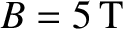(low-field) or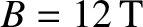(high-field),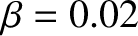,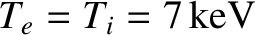,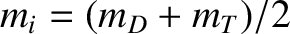(where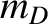and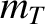are the deuteron and triton masses, respectively),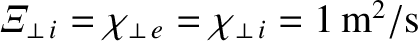,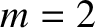,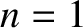,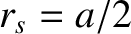(where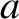is the minor radius of the plasma),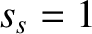,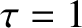,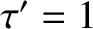,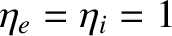, and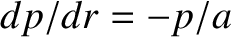.

Table 11.1: Parameters characterizing the rescaled reduced neoclassical drift-MHD equations for a low-field and a high-field tokamak reactor. [See Equations (11.6), (11.74), (11.75), and (11.80)–(11.82).]
 Low-Field High-Field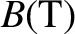5.0 12.0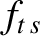0.565 0.565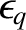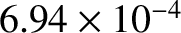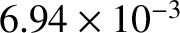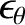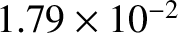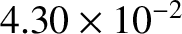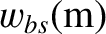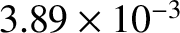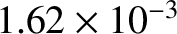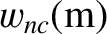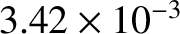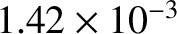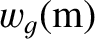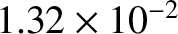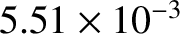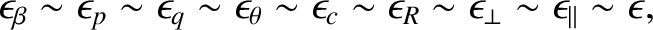(11.92)

where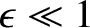. As is clear from Tables 5.1 and 11.1, these orderings are reasonable provided that the radial width of the magnetic island chain that develops in the inner region exceeds a few centimeters.

Let us also adopt the orderings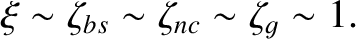(11.93)

As is clear from Tables 5.1 and 11.1, the ordering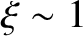is consistent with the orderings (11.92). Unfortunately, the orderings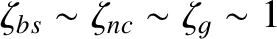are not consistent with the orderings (11.92). (It would actually be more consistent to adopt the orderings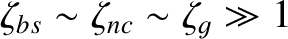.) Nevertheless, we are forced to adopt the orderingsin order to make further progress. (By adopting these ordering we are essentially ensuring that the bootstrap current and curvature terms in our analysis are not so large that they lead to a breakdown of the constant-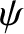approximation.)

Finally, suppose that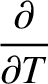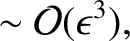(11.94)

and let us expand the various fields in our rescaled model as follows: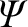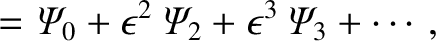(11.95)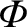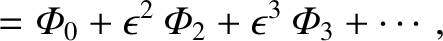(11.96)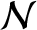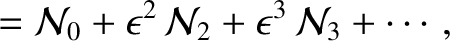(11.97)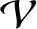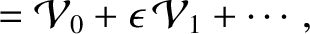(11.98)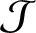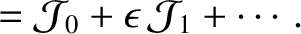(11.99)

Here,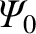,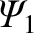, et cetera are assumed to be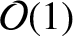in the inner region.